# Centre manifold

(diff) ← Older revision | Latest revision (diff) | Newer revision → (diff)

Consider an autonomous system of ordinary differential equations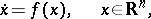(a1)

where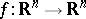is sufficiently smooth,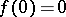. Let the eigenvalues of the Jacobi matrixevaluated at the equilibrium position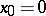be. Suppose the equilibrium is non-hyperbolic, i.e. has eigenvalues with zero real part. Assume also that there are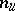eigenvalues (counting multiplicities) with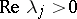,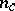eigenvalues with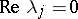, and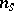eigenvalues with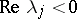. Let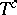denote the linear (generalized) eigenspace ofcorresponding to the union of theeigenvalues on the imaginary axis. The eigenvalues withare often called critical, as is the eigenspace. Letdenote the flow (continuous-time dynamical system) associated with (a1). Under the assumptions stated above, the following centre manifold theorem holds [a7], [a9], [a3], [a11]: There is a locally defined smooth-dimensional invariant manifold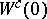of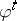that is tangent toat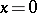.

The manifoldis called the centre manifold. The centre manifoldneed not be unique. If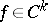with finite,is a-manifold in some neighbourhoodof. However, as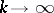the neighbourhoodmay shrink, thus resulting in the non-existence of a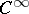-manifoldfor certainsystems.

In a basis formed by all (generalized) eigenvectors of(or their linear combinations if the corresponding eigenvalues are complex), the system (a1) can be written as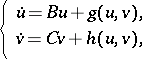(a2)

where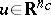,,is an-matrix with all itseigenvalues on the imaginary axis, whileis an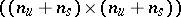-matrix with no eigenvalue on the imaginary axis;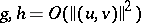. A centre manifold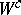of (a2) can be locally represented as the graph of a smooth function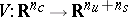,: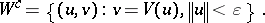The following reduction principle is valid (see [a1], [a8]): The system (a2) is locally topologically equivalent (cf. Equivalence of dynamical systems) near the origin to the system(a3)

The equations forandare uncoupled in (a3). The first equation is the restriction of (a2) to its centre manifold. Thus, the dynamics of (a2) near a non-hyperbolic equilibrium are determined by this restriction, since the second equation in (a3) is linear and has exponentially decaying/growing solutions. For example, if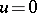is the asymptotically stable equilibrium of the restriction and the matrixhas no eigenvalue with positive real part, then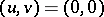is the asymptotically stable equilibrium of (a2). If there is more than one centre manifold, then all the resulting systems (a3) with differentare locally topologically equivalent (actually, thediffer only by flat functions).

The second equation in (a3) can be replaced by the standard saddle: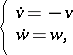(a4)

with. In other words, near a non-hyperbolic equilibrium the system is locally topologically equivalent to the suspension of its restriction to the centre manifold by the standard saddle.

Consider now a system that depends smoothly on parameters: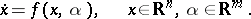(a5)

Suppose that at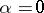the system has a non-hyperbolic equilibriumwitheigenvalues on the imaginary axis and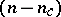eigenvalues with non-zero real part. Letof them have negative real part, whilehave positive real part. Applying the centre manifold theorem to the following extended system:(a6)

one can prove the existence of a parameter-dependent local invariant manifold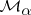in (a5). The manifold has dimensionand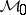coincides with a centre manifoldof the (a5) at. Often, the manifoldis called the centre manifold for all. For each small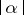one can restrict system (a5) to. Introducing a coordinate system onwithas the coordinate, this restriction will be represented by a smooth system: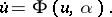(a7)

At, the system (a7) is equivalent to the restriction of (a5) to its centre manifold. The following results are known as the Šošitaišvili theorem [a10] (see also [a1], [a2], [a8]): The system (a5) is locally topologically equivalent near the origin to the suspension of (a7) by the standard saddle (a4). Moreover, (a7) can be replaced by any locally topologically equivalent system.

This theorem reduces the study of bifurcations of non-hyperbolic equilibria (cf. also Bifurcation) to those on the corresponding centre manifold of dimension equal to the number of critical eigenvalues. There are analogues of the reduction principle and Šošitaišvili's theorem for discrete-time dynamical systems defined by iterations of diffeomorphisms (see, for example, [a1], [a8]). Existence of centre manifolds has also been proved for certain infinite-dimensional dynamical systems defined by partial differential equations [a9], [a3], [a6] and delay differential equations [a5], [a4].

How to Cite This Entry:
Centre manifold. Encyclopedia of Mathematics. URL: http://encyclopediaofmath.org/index.php?title=Centre_manifold&oldid=16865
This article was adapted from an original article by Yu.A. Kuznetsov (originator), which appeared in Encyclopedia of Mathematics - ISBN 1402006098. See original article